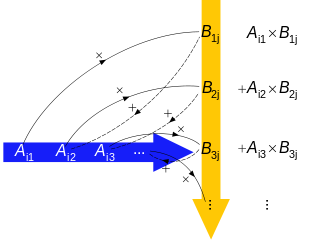## Matrix multiplication Calculator

Matrix 1 x Matrix 2
Matrix Type x Matrix Type
x
=
=

The matrix multiplication is matrix A multiplied by another matrix B, You can follow the step Fig below:Arithmetic process of multiplying numbers (solid lines) in row i in matrix A and column j in matrix B, then adding the terms (dashed lines) to obtain entry ij in the final matrix.

To the calculator, Select the matrix 1 type and the matrix 2 type, Enter the proper number click the "calculate" button get the resutl.

To the matrix multiplication the two matrix only when the number of columns of first matrix is equal to number of rows of second matrix.

Thinkcalculator.com provides you helpful and handy calculator resources.